f
Neutrium

# Welcome to Neutrium

Neutrium is a knowledge base of engineering topics, centred mainly around chemical engineering design challenges faced by engineers in their daily work. We created Neutrium to bridge the gap between theory and practice. Feel free to ask a question, leave feedback or take a look at one of our in-depth articles.

Packed Bed Reynolds Number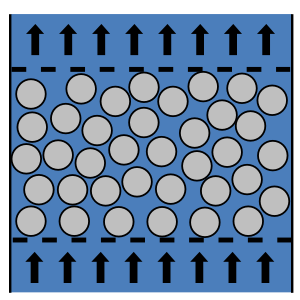The packed bed Reynolds number is dimensionless and describes the ratio of inertial to viscous forces for fluid flow through a packed bed. It may be used to calculate the pressure drop though a packed bed via the Ergun equation or identify the boundaries of flow regimes (laminar, transitional and turbulent) in a packed bed. This article will show you how to calculate and interpret the packed bed Reynolds number.

Ideal Gas Law

The ideal gas law is used to relate volume, pressure and temperature for gases. The ideal gas law is an approximation and is generally more accurate at mild temperatures and pressures. The properties of gases increasingly deviate from ideal as conditions become more extreme, i.e. low temperature or high pressure. In these cases a compressibility factor may be used to correct for non-ideal behaviour when using the ideal gas relationship.

Thermal Resistance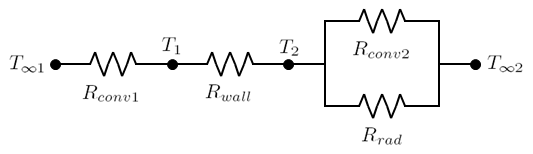The concept of thermal resistance can be utilised to solve steady state heat transfer problems that involve series, parallel or combined series-parallel components. This article demonstrates how to calculate the total thermal resistance for such systems and how to calculate the thermal resistance for practical geometries such as a pipe wall.

Prandtl Number

The Prandtl number is a dimensionless number named after the German physicist Ludwig Prandtl. It represents the ratio of molecular diffusivity of momentum to the molecular diffusivity of heat.

Conversion of Standard Volumetric Flow Rates of Gas

Standard volumetric flow rates of a fluid are the equivalent of actual volumetric flow rates in the sense that they have an equal mass flow rate. This identity makes standard volumetric flow appropriate providing a common baseline for comparison of volumetric gas flow rate measurements at different conditions. This article outlines how to convert between standard and actual volumetric flow rates.

Absolute Roughness of Pipe MaterialAbsolute roughness is a measure of the surface roughness of a material which a fluid may flow over. Absolute roughness is important when calculating pressure drop particularly in the turbulent flow regime. This article provides some typical absolute roughness values for common conduit materials.

Friction Factor for Flow in Coils and Curved Pipe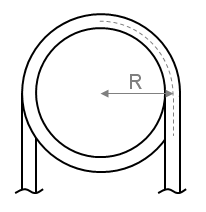In order to determine the pressure drop in a pipe or coil the friction factor must first be calculated. This article presents the equations which may be used to determine the friction factor in coils and curved pipe.

Pressure Loss Cv and Kv Method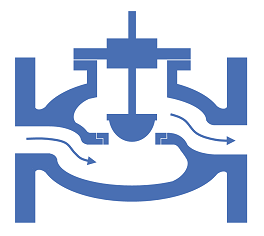Cv and Kv are singles values in units of flowrate that may be used to characterise the relationship between flowrate and pressure loss for fittings and equipment. This article demonstrates how to calculate the Cv or Kv values, and how to use these values to determine the pressure loss for a given flowrate.

Pressure Loss in Pipe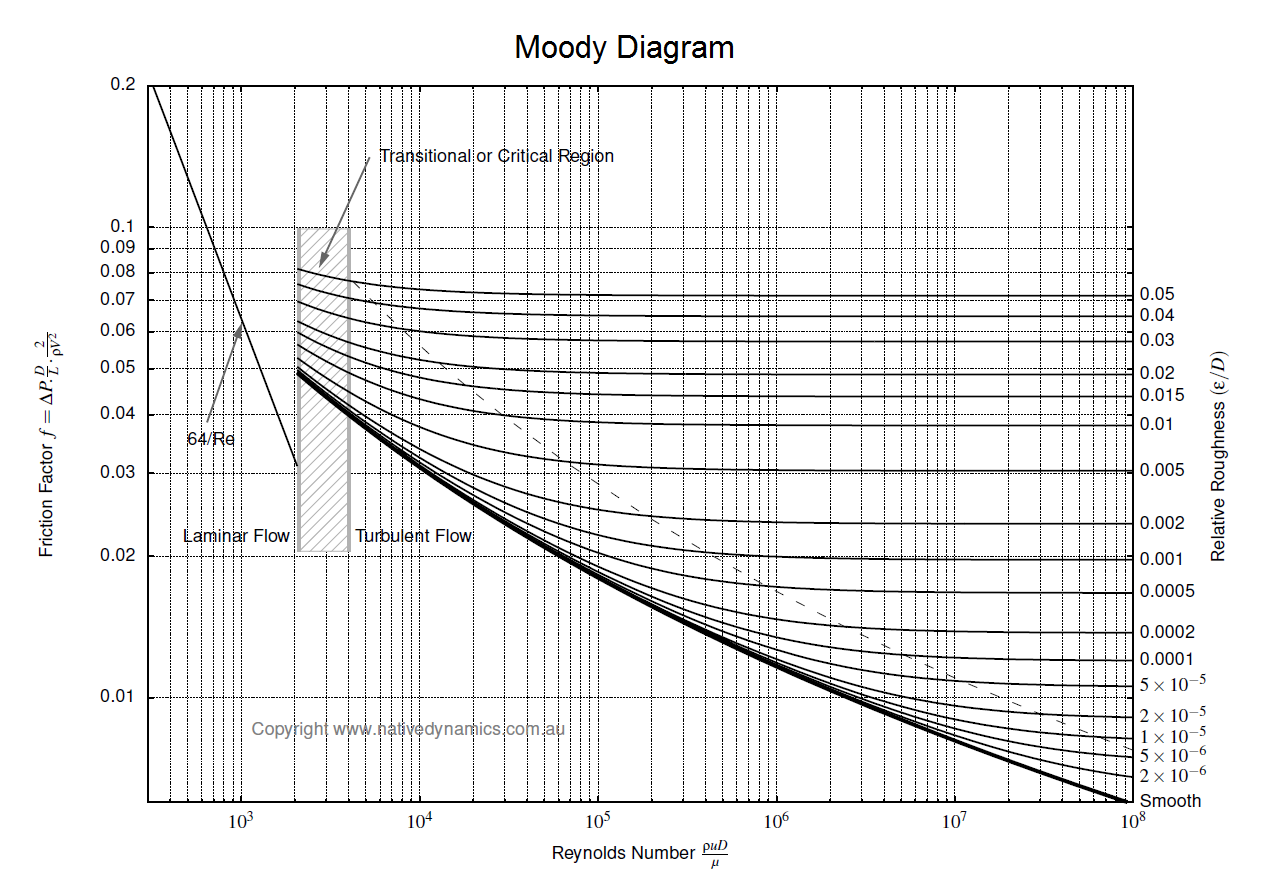To determine the pressure loss or flow rate through pipe knowledge of the friction between the fluid and the pipe is required. This article describes how to incorporate friction into pressure loss or fluid flow calculations. It also outlines several methods for determining the Darcy friction factor for rough and smooth pipes in both the turbulent and laminar flow regime. Finally this article discusses which correlation for pressure loss in pipe is the most appropriate.

Pump Cavitation and NPSH

Cavitation is the formation and subsequent collapse of vapour bubbles in a flowing liquid and is often responsible for significant damage flow equipment such as pumps. This article will provide an overview of cavitation and provide insight into the identification and prevention of cavitation conditions through the calculation of Net Positive Suction Head (NPSH).